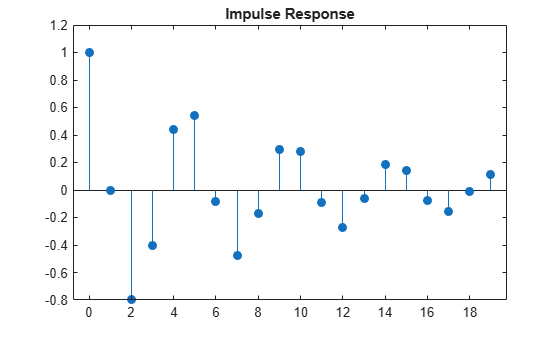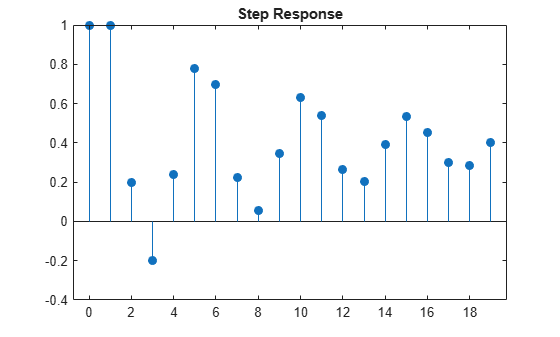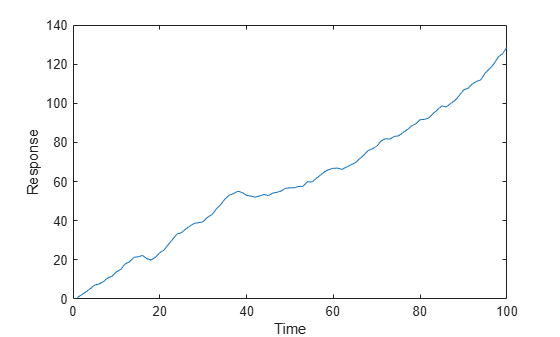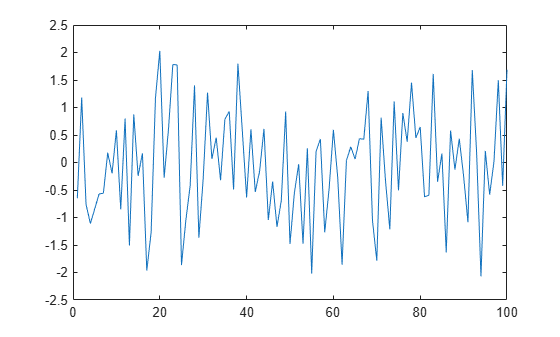# filter

Filter disturbances using ARIMA or ARIMAX model

## Syntax

``Y = filter(Mdl,Z)``
``Y = filter(Mdl,Z,Name,Value)``
``````[Y,E] = filter(___)``````
``````[Y,E,V] = filter(___)``````

## Description

example

````Y = filter(Mdl,Z)` returns the response series `Y` resulting from filtering the underlying disturbance series `Z`. `Z` is associated with the model innovations process through the ARIMA model `Mdl`.```

example

````Y = filter(Mdl,Z,Name,Value)` uses additional options specified by one or more name-value arguments. For example, `filter(Mdl,Z,'X',X,'Z0',Z0)` specifies exogenous predictor data `X` and presample disturbances `Z0` to initialize the model.```

example

``````[Y,E] = filter(___)``` returns the model innovations series `E` using any of the input arguments in the previous syntaxes.```

example

``````[Y,E,V] = filter(___)``` returns the conditional variances `V` for a composite conditional mean and variance model (for example, an ARIMA and GARCH composite model).```

## Examples

collapse all

Specify a mean zero ARIMA(2,0,1) model.

```Mdl = arima('Constant',0,'AR',{0.5,-0.8},'MA',-0.5,... 'Variance',0.1);```

Simulate the first 20 responses of the impulse response function (IRF). Generate a disturbance series with a one-time, unit impulse, and then filter it.

```z = [1; zeros(19,1)]; y = filter(Mdl,z);```

`y` is a 20-by-1 response path resulting from filtering the disturbance path `z` through the model. y represents the IRF. The `filter` function requires one presample observation to initialize the model. By default, `filter` uses the unconditional mean of the process, which is `0`.

`y = y/y(1);`

Normalize the IRF such that the first element is 1.

Plot the impulse response function.

```figure; stem((0:numel(y)-1)',y,'filled'); title 'Impulse Response';```The impulse response assesses the dynamic behavior of a system to a one-time, unit impulse.

Alternatively, you can use the `impulse` function to plot the IRF for an ARIMA process.

Assess the dynamic behavior of a system to a persistent change in a variable by plotting a step response.

Specify a mean zero ARIMA(2,0,1) process.

```Mdl = arima('Constant',0,'AR',{0.5,-0.8},'MA',-0.5,... 'Variance',0.1);```

Simulate the first 20 responses to a sequence of unit disturbances. Generate a disturbance series of ones, and then filter it. Set all presample observations equal to zero.

```Z = ones(20,1); Y = filter(Mdl,Z,'Y0',zeros(Mdl.P,1)); Y = Y/Y(1);```

The last step normalizes the step response function to ensure that the first element is 1.

Plot the step response function.

```figure; stem((0:numel(Y)-1)',Y,'filled'); title 'Step Response';```Create models for the response and predictor series. Set an ARIMAX(2,1,3) model to the response `MdlY`, and an AR(1) model to the `MdlX`.

```MdlY = arima('AR',{0.1 0.2},'D',1,'MA',{-0.1 0.1 0.05},... 'Constant',1,'Variance',0.5, 'Beta',2); MdlX = arima('AR',0.5,'Constant',0,'Variance',0.1);```

Simulate a length 100 predictor series `x` and a series of iid normal disturbances `z` having mean zero and variance 1.

```rng(1); z = randn(100,1); x = simulate(MdlX,100);```

Filter the disturbances `z` using `MdlY` to produce the response series `y`, and plot `y`.

```y = filter(MdlY,z,'X',x); figure; plot(y); title 'Filter to simulate ARIMA(2,1,3)'; xlabel 'Time'; ylabel 'Response';```Create a mean zero ARIMA(2,0,1) model.

```Mdl = arima('Constant',0,'AR',{0.5,-0.8},'MA',-0.5,... 'Variance',0.1);```

Generate 20 random paths from the model.

```rng(1); % For reproducibility [ySim,eSim,vSim] = simulate(Mdl,100,'NumPaths',20);```

`ySim`, `eSim`, and `vSim` are 100-by-20 matrices of 20 simulated response, innovation, and conditional variance paths of length 100, respectively. Because Mdl does not have a conditional variance model, `vSim` is a matrix completely composed of the value of `Mdl.Variance`.

Obtain disturbance paths by standardizing the simulated innovations.

`zSim = eSim./sqrt(vSim);`

Filter the disturbance paths through the model.

`[yFil,eFil] = filter(Mdl,zSim);`

`yFil` and `eFil` are 100-by-20 matrices. The columns are independent paths generated from filtering corresponding disturbance paths in `zSim` through the model `Mdl`.

Confirm that the outputs of `simulate` and `filter` are identical.

`sameE = norm(eSim - eFil) <eps`
```sameE = logical 1 ```
`sameY = norm(ySim - yFil) < eps`
```sameY = logical 1 ```

The logical values `1` confirm the outputs are effectively identical.

Create the composite AR(1)/GARCH(1,1) model

`$\begin{array}{l}{y}_{t}=1+0.5{y}_{t-1}+{\epsilon }_{t}\\ {\epsilon }_{t}={\sigma }_{t}{z}_{t}\\ {\sigma }_{t}^{2}=0.2+0.1{\sigma }_{t-1}^{2}+0.05{\epsilon }_{t-1}^{2}\\ {z}_{t}\sim N\left(0,1\right).\end{array}$`

Create the composite model.

```CVMdl = garch('Constant',0.2,'GARCH',0.1,'ARCH',0.05); Mdl = arima('Constant',1,'AR',0.5,'Variance',CVMdl)```
```Mdl = arima with properties: Description: "ARIMA(1,0,0) Model (Gaussian Distribution)" Distribution: Name = "Gaussian" P: 1 D: 0 Q: 0 Constant: 1 AR: {0.5} at lag  SAR: {} MA: {} SMA: {} Seasonality: 0 Beta: [1×0] Variance: [GARCH(1,1) Model] ```

`Mdl` is an `arima` object. The property `Mdl.Variance` contains a `garch` object that represents the conditional variance model.

Generate a random series of 100 standard Gaussian of disturbances.

```rng(1) % For reproducibility z = randn(100,1);```

Filter the disturbances through the model. Return and plot the simulated conditional variances.

```[y,e,v] = filter(Mdl,z); plot(z)```## Input Arguments

collapse all

Fully specified ARIMA model, specified as an `arima` model object created by `arima` or `estimate`.

The properties of `Mdl` cannot contain `NaN` values.

Underlying disturbance series zt, specified as a length `numObs` numeric column vector or a `numObs`-by-`numPaths` numeric matrix. `numObs` is the length of the time series (sample size). `numPaths` is the number of separate, independent disturbance paths.

zt drives the innovation process εt. For a variance process σt2, the innovation process is

`${\epsilon }_{t}={\sigma }_{t}{z}_{t}.$`

Each row corresponds to a period. The last row contains the latest set of disturbances.

Each column corresponds to a separate, independent path of disturbances. `filter` assumes that disturbances across any row occur simultaneously.

`Z` is the continuation of the presample disturbances `Z0`.

Data Types: `double`

### Name-Value Arguments

Specify optional pairs of arguments as `Name1=Value1,...,NameN=ValueN`, where `Name` is the argument name and `Value` is the corresponding value. Name-value arguments must appear after other arguments, but the order of the pairs does not matter.

Before R2021a, use commas to separate each name and value, and enclose `Name` in quotes.

Example: `filter(Mdl,Z,'X',X,'Z0',Z0)` specifies exogenous predictor data `X` and presample disturbances `Z0` to initialize the model.

Presample response data providing initial values for the model, specified as a numeric column vector or a numeric matrix.

Each row of `Y0` corresponds to a period in the presample. The following conditions apply:

• The last row contains the latest presample responses.

• To initialize the AR components, `Y0` must have at least `Mdl.P` rows.

• If `Y0` has more rows than is required to initialize the model, `filter` uses only the latest required rows.

Columns of `Y0` are separate, independent presample paths. The following conditions apply:

• If `Y0` is a column vector, `filter` applies it to each path.

• If `Y0` is a matrix, `filter` applies `Y0(:,j)` to initialize path `j`. `Y0` must have at least `numPaths` columns; `filter` uses only the first `numPaths` columns of `Y0`.

By default, `filter` sets any necessary presample observations by using one of the following methods:

• For a model with a stationary AR process and without a regression component, `filter` sets all presample responses to the unconditional mean of the model.

• For a model that represents a nonstationary process or that contains a regression component, `filter` sets all necessary presample responses to zero.

Data Types: `double`

Presample disturbances providing initial values for the input disturbance series `Z`, specified as a numeric column vector or a numeric matrix.

Each row of `Z0` corresponds to a period in the presample. The following conditions apply:

• The last row contains the latest presample disturbances.

• To initialize the MA components, `Z0` must have at least `Mdl.Q` rows.

• If `Mdl.Variance` is a conditional variance model (for example, a `garch` model object), `Z0` can require more rows than `Mdl.Q` to initialize the model.

• If `Z0` has more rows than is required to initialize the model, `filter` uses only the latest required rows.

Columns of `Z0` are separate, independent presample paths. The following conditions apply:

• If `Z0` is a column vector, `filter` applies it to each path.

• If `Z0` is a matrix, `filter` applies `Z0(:,j)` to initialize path `j`. `Z0` must have at least `numPaths` columns; `filter` uses only the first `numPaths` columns of `Z0`.

By default, `filter` sets the necessary presample disturbances to 0.

Data Types: `double`

Presample conditional variance data used to initialize the conditional variance model, specified as a positive numeric column vector or a positive numeric matrix. If the conditional variance `Mdl.Variance` is constant, `filter` ignores `V0`.

Each row of `V0` corresponds to a period in the presample. The following conditions apply:

• The last row contains the latest presample conditional variances.

• To initialize the conditional variance model, `V0` must have at least `Mdl.Q` rows. For details, see the `filter` function of conditional variance models.

• If `V0` has more rows than is required to initialize the conditional variance model, `filter` uses only the latest required rows.

Columns of `V0` are separate, independent presample paths. The following conditions apply:

• If `V0` is a column vector, `filter` applies it to each simulated path.

• If `V0` is a matrix, `filter` applies `V0(:,j)` to initialize simulating path `j`. `V0` must have at least `numPaths` columns; `filter` uses only the first `numPaths` columns of `V0`.

By default, `filter` sets all necessary presample observations to the unconditional variance of the conditional variance process.

Data Types: `double`

Exogenous predictor data for the regression component in the model, specified as a `numObs`-by-`numPreds` numeric matrix.

`numPreds` is the number of predictor variables (`numel(Mdl.Beta)`).

Each row of `X` corresponds to the corresponding period in `Z` (period for which `filter` filters disturbances; the period after the presample). The following conditions apply:

• The last row contains the latest predictor data.

• If the specified predictor data has more then `numObs` rows, `filter` uses only the latest `numObs` rows.

• `filter` does not use the regression component in the presample period.

Columns of `X` are separate predictor variables.

`filter` applies `X` to each filtered path; that is, `X` represents one path of observed predictors.

By default, `filter` excludes the regression component, regardless of its presence in `Mdl`.

Data Types: `double`

Note

• `NaN`s in input data indicate missing values. `filter` uses listwise deletion to delete all sampled times (rows) in the input data containing at least one missing value. Specifically, `filter` performs these steps:

1. Synchronize, or merge, the presample data sets `Y0`, `Z0`, and `V0` to create the set `Presample`, in other words, `Presample` = `[Y0 Z0 V0]`.

2. Synchronize the filter data `Z` and `X` to create the set `Data`, in other words, `Data` = `[Z X]`.

3. Remove all rows from `Presample` and `Data` containing at least one `NaN`.

Listwise deletion applied to the filter data can reduce the sample size and create irregular time series.

`filter` merges data sets that have a different number of rows according to the latest observation, and then pads the shorter series with enough default values to form proper matrices.

• `filter` assumes that you synchronize the data sets so that the latest observations occur simultaneously. The software also assumes that you synchronize the presample series similarly.

## Output Arguments

collapse all

Simulated response paths yt, returned as a length `numObs` column vector or a `numObs`-by-`numPaths` numeric matrix.

For each `t` = 1, …, `numObs`, the simulated response at time `t` `Y(t,:)` corresponds to the filtered disturbance at time `t` `Z(t,:)` and response path `j` `Y(:,j)` corresponds to the filtered disturbance path `j` `Z(:,j)`.

`Y` represents the continuation of the presample response paths in `Y0`.

Simulated paths of model innovations εt with conditional variances ${\sigma }_{t}^{2}$ (`V`), returned as a length `numObs` column vector or a `numObs`-by-`numPaths` numeric matrix. The dimensions of `Y` and `E` correspond.

Columns of `E` are scaled disturbance paths (innovations) such that, for a particular path

`${\epsilon }_{t}={\sigma }_{t}{z}_{t}.$`

Conditional variance paths ${\sigma }_{t}^{2}$, returned as a length `numObs` column vector or `numObs`-by-`numPaths` numeric matrix. The dimensions of `Y` and `V` correspond.

If `Z` is a matrix, then the columns of `V` are the filtered conditional variance paths corresponding to the columns of `Z`.

Columns of `V` are conditional variance paths of corresponding paths of innovations εt (`E`) such that, for a particular path

`${\epsilon }_{t}={\sigma }_{t}{z}_{t}.$`

`V` represents the continuation of the presample conditional variance paths in `V0`.

## Alternative Functionality

`filter` generalizes `simulate`; both functions filter a series of disturbances to produce output responses, innovations, and conditional variances. However, `simulate` autogenerates a series of mean zero, unit variance, independent and identically distributed (iid) disturbances according to the distribution in `Mdl`. In contrast, `filter` enables you to directly specify custom disturbances.

 Box, George E. P., Gwilym M. Jenkins, and Gregory C. Reinsel. Time Series Analysis: Forecasting and Control. 3rd ed. Englewood Cliffs, NJ: Prentice Hall, 1994.

 Enders, Walter. Applied Econometric Time Series. Hoboken, NJ: John Wiley & Sons, Inc., 1995.

 Hamilton, James D. Time Series Analysis. Princeton, NJ: Princeton University Press, 1994.